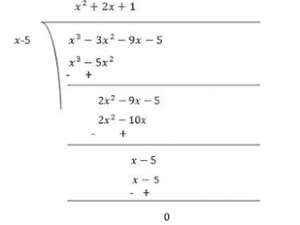Guru

# Factorize:(ii) x3–3×2–9x–5 Q.5(2)

• 0

I don’t know how to solve this problem in simple way of Polynomials chapter of exercise 2.4 of class 9th math. Give me the best answer of this question  Factorize:(ii) x3–3×2–9x–5

Share

1. Let p(x) = x3–3x2–9x–5

Factors of 5 are ±1 and ±5

By trial method, we find that

p(5) = 0

So, (x-5) is factor of p(x)

Now,

p(x) = x3–3x2–9x–5

p(5) = (5)3–3(5)2–9(5)–5

= 125−75−45−5

= 0

Therefore, (x-5) is the factor of  p(x)Now, Dividend = Divisor × Quotient + Remainder

(x−5)(x2+2x+1) = (x−5)(x2+x+x+1)

= (x−5)(x(x+1)+1(x+1))

= (x−5)(x+1)(x+1)

• 0